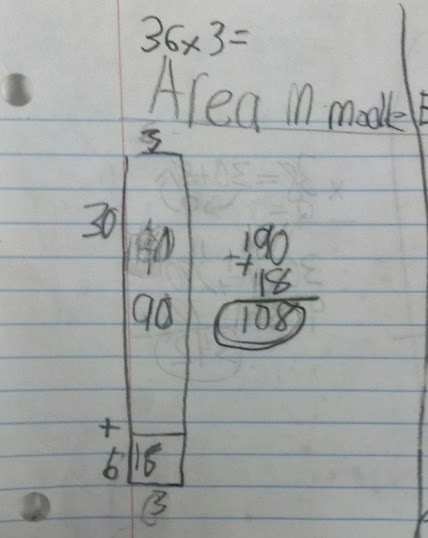# Multiplying one digit by two digits using the AREA MODEL

45 teachers like this lesson
Print Lesson

## Objective

SWBAT find products of one digit by two digit multiplication using the area model.

#### Big Idea

Students make connections between the area model and multiplying by multiples of ten while deepening their understanding of multiplication.

## Warm Up

5 minutes

This is a quick review from yesterday's lesson, multiplying by multiples of ten. I display these problems on the board one at a time.

60 x _____= 360

350 = 70 x _____

900= 100 x ____

80 x _____ = 640

## Concept development

45 minutes

This lesson should look familiar to a lesson I tried last week.  It became obvious during that lesson that students needed more guidance and scaffolds before they could be successful with this lesson. (In a way this is take two of a previous lesson.)

I begin this by modeling the area model for multiplication using a multiple of ten.  Since my students did so well with multiplying multiples of ten in yesterday's lesson, I wanted to incorporate that and note that with an area model. I show this by writing a number sentence like 6 x 70 on the smartboard.  Then I draw a long horizontal rectangle to model an area of 6 rows by 70 columns.  I make explicit that in the number 70, that is 7 tens and NO ones.  I find this language crucial for students to see the relationship between what they did when multiplying by multiples of ten and then transferring that knowledge to other double digit numbers today.

Then I show an area model for a two digit by one-digit numbers sentence. I model this by decomposing a 2-digit number into tens and ones, and then model area representations  and partial product arrays. So, for example, 63 x 7 can be modeled with 60 + 3 multiplied by 7 columns.

If you're not sure what the area model for multiplication is, you can watch the showme video below.

This lesson provides conceptual understanding of what occurs in a 2-digit by one-digit multiplication problem and allows students to deepen their understanding of multiplication as they build their skills in order to master CCSS 4.NBT.5. Partial product models and area models serve as transitions to understanding the standard multiplication algorithm.

After I show the area model, we do several together.  Students build the area models on  small graph paper. They build the rectangles, using the correct amount of squares to visually see the square units. I find this step to be necessary before using a paper and pencil model. As students use the graph paper, I discuss with them that the paper doesn't have enough rows or columns of squares to make large models.  This serves as a springboard to then discuss how the model, "models" the situation and does not have to be drawn to scale.

Then I have students participate in an activity I call "Walk the Room."

Students will use clipboards as they walk around the room to solve the problems that are posted.  When students did this before, they solved problems independently. I decided to have students use learning partners because I want to continue to foster their communication skills in order to develop Math Practice Standard 3.  The partner work allows for opportunities to communicate with the area model and talk through problems with a peer.  In the photo below, you can see a sample of a students area model.I have many two-digit by one-digit multiplication number sentences hanging around the room for students to solve. They do not need to solve all of them, there are a lot. I tell students that I would like theme to solve between 4and 7 problems. Students can wander to a problem that is not being completed by another student. See this video for what my classroom looked like.

The area model is the easiest method for students to use.  By teaching it first, I am able to build conceptual understanding for all students and provide a method that any student can use. For students that struggle with the area model, I pull them out during my re-teach time and present other alternatives, such as using base ten blocks.  Students would build a number like 26 and then do that 4 times to model 4 x 26.  I then make connections between the base ten block method and the area model method by rearranging the base ten blocks to show the two columns of tens together in four rows and the  six columns of ones together in four rows.

## Student debrief - Wrap up

15 minutes

Students spend the last 10 minutes of the lesson making a poster with their learning partner. Their poster must meet these requirements:

1.  Choose a single digit by double digit multiplication problem to show on your poster.  Write it BIG so people can see it from far away, but not too big.  Your poster will have other items on it as well.

2.  Show an AREA MODEL for your problem.

3.  Using words, pictures and numbers communicate how to use an area model to find products.

Students do not have time to present their posters to the class during this time period, but as they are working on their posters, I am able to talk with partners about their posters.  This is a great time to see if anyone has misconceptions that need cleared up.  This is a busy and active time in my classroom. I have found that playing low classical music keeps the noise level low and students more on task.  I do like reminding them about not being sheep or hogs from the partner video we've watched before.

These two students explain multiplying 46 x 9 in this video as they make their poster.